# Kepler Problem

##### Yingbo Ma, Chris Rackauckas

The Hamiltonian $\mathcal {H}$ and the angular momentum $L$ for the Kepler problem are

$\mathcal {H} = \frac{1}{2}(\dot{q}^2_1+\dot{q}^2_2)-\frac{1}{\sqrt{q^2_1+q^2_2}},\quad L = q_1\dot{q_2} - \dot{q_1}q_2$

Also, we know that

${\frac {\mathrm {d} {\boldsymbol {p}}}{\mathrm {d} t}}=-{\frac {\partial {\mathcal {H}}}{\partial {\boldsymbol {q}}}}\quad ,\quad {\frac {\mathrm {d} {\boldsymbol {q}}}{\mathrm {d} t}}=+{\frac {\partial {\mathcal {H}}}{\partial {\boldsymbol {p}}}}}$

using OrdinaryDiffEq, LinearAlgebra, ForwardDiff, Plots; gr()
H(q,p) = norm(p)^2/2 - inv(norm(q))
L(q,p) = q*p - p*q

pdot(dp,p,q,params,t) = ForwardDiff.gradient!(dp, q->-H(q, p), q)
qdot(dq,p,q,params,t) = ForwardDiff.gradient!(dq, p-> H(q, p), p)

initial_position = [.4, 0]
initial_velocity = [0., 2.]
initial_cond = (initial_position, initial_velocity)
initial_first_integrals = (H(initial_cond...), L(initial_cond...))
tspan = (0,20.)
prob = DynamicalODEProblem(pdot, qdot, initial_velocity, initial_position, tspan)
sol = solve(prob, KahanLi6(), dt=1//10);


Let's plot the orbit and check the energy and angular momentum variation. We know that energy and angular momentum should be constant, and they are also called first integrals.

plot_orbit(sol) = plot(sol,vars=(3,4), lab="Orbit", title="Kepler Problem Solution")

function plot_first_integrals(sol, H, L)
plot(initial_first_integrals.-map(u->H(u[2,:], u[1,:]), sol.u), lab="Energy variation", title="First Integrals")
plot!(initial_first_integrals.-map(u->L(u[2,:], u[1,:]), sol.u), lab="Angular momentum variation")
end
analysis_plot(sol, H, L) = plot(plot_orbit(sol), plot_first_integrals(sol, H, L))

analysis_plot (generic function with 1 method)

analysis_plot(sol, H, L)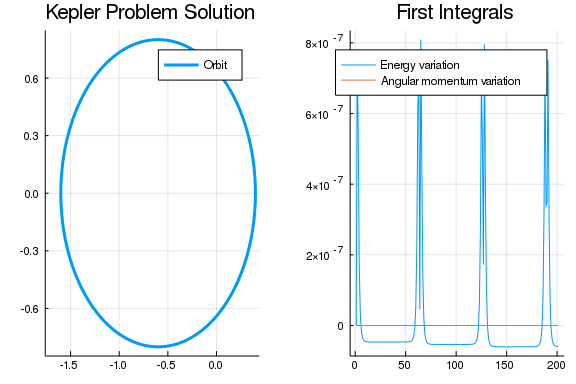Let's try to use a Runge-Kutta-Nyström solver to solve this problem and check the first integrals' variation.

sol2 = solve(prob, DPRKN6())  # dt is not necessary, because unlike symplectic
@show sol2.u |> length

sol2.u |> length = 79

analysis_plot(sol2, H, L)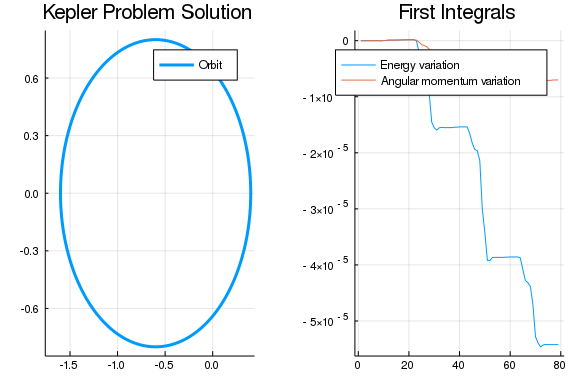Let's then try to solve the same problem by the ERKN4 solver, which is specialized for sinusoid-like periodic function

sol3 = solve(prob, ERKN4()) # dt is not necessary, because unlike symplectic
@show sol3.u |> length

sol3.u |> length = 52

analysis_plot(sol3, H, L)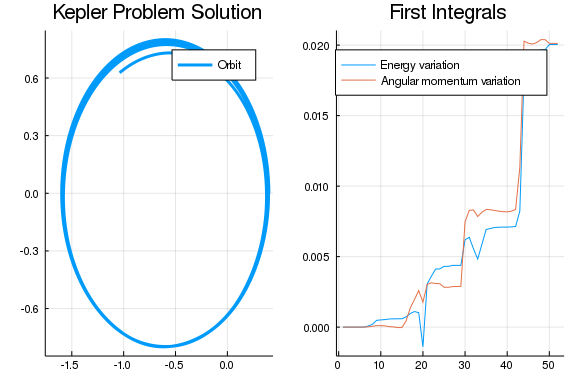We can see that ERKN4 does a bad job for this problem, because this problem is not sinusoid-like.

One advantage of using DynamicalODEProblem is that it can implicitly convert the second order ODE problem to a normal system of first order ODEs, which is solvable for other ODE solvers. Let's use the Tsit5 solver for the next example.

sol4 = solve(prob, Tsit5())
@show sol4.u |> length

sol4.u |> length = 54

analysis_plot(sol4, H, L)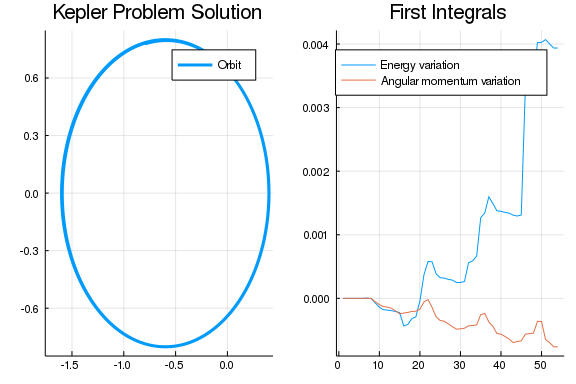#### Note

There is drifting for all the solutions, and high order methods are drifting less because they are more accurate.

### Conclusion

Symplectic integrator does not conserve the energy completely at all time, but the energy can come back. In order to make sure that the energy fluctuation comes back eventually, symplectic integrator has to have a fixed time step. Despite the energy variation, symplectic integrator conserves the angular momentum perfectly.

Both Runge-Kutta-Nyström and Runge-Kutta integrator do not conserve energy nor the angular momentum, and the first integrals do not tend to come back. An advantage Runge-Kutta-Nyström integrator over symplectic integrator is that RKN integrator can have adaptivity. An advantage Runge-Kutta-Nyström integrator over Runge-Kutta integrator is that RKN integrator has less function evaluation per step. The ERKN4 solver works best for sinusoid-like solutions.

## Manifold Projection

In this example, we know that energy and angular momentum should be conserved. We can achieve this through mainfold projection. As the name implies, it is a procedure to project the ODE solution to a manifold. Let's start with a base case, where mainfold projection isn't being used.

using DiffEqCallbacks

plot_orbit2(sol) = plot(sol,vars=(1,2), lab="Orbit", title="Kepler Problem Solution")

function plot_first_integrals2(sol, H, L)
plot(initial_first_integrals.-map(u->H(u[1:2],u[3:4]), sol.u), lab="Energy variation", title="First Integrals")
plot!(initial_first_integrals.-map(u->L(u[1:2],u[3:4]), sol.u), lab="Angular momentum variation")
end

analysis_plot2(sol, H, L) = plot(plot_orbit2(sol), plot_first_integrals2(sol, H, L))

function hamiltonian(du,u,params,t)
q, p = u[1:2], u[3:4]
qdot(@view(du[1:2]), p, q, params, t)
pdot(@view(du[3:4]), p, q, params, t)
end

prob2 = ODEProblem(hamiltonian, [initial_position; initial_velocity], tspan)
sol_ = solve(prob2, RK4(), dt=1//5, adaptive=false)
analysis_plot2(sol_, H, L)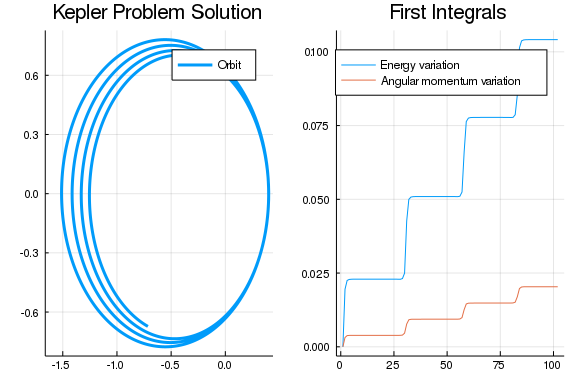There is a significant fluctuation in the first integrals, when there is no mainfold projection.

function first_integrals_manifold(residual,u)
residual[1:2] .= initial_first_integrals - H(u[1:2], u[3:4])
residual[3:4] .= initial_first_integrals - L(u[1:2], u[3:4])
end

cb = ManifoldProjection(first_integrals_manifold)
sol5 = solve(prob2, RK4(), dt=1//5, adaptive=false, callback=cb)
analysis_plot2(sol5, H, L)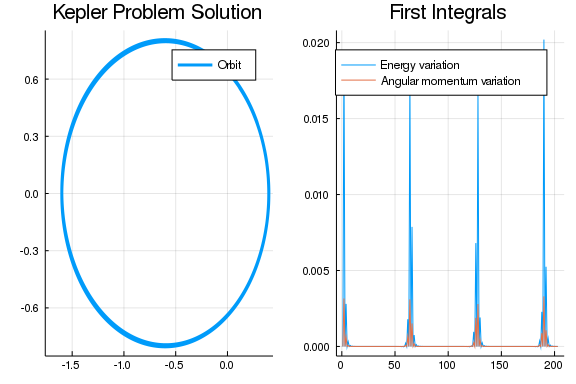We can see that thanks to the manifold projection, the first integrals' variation is very small, although we are using RK4 which is not symplectic. But wait, what if we only project to the energy conservation manifold?

function energy_manifold(residual,u)
residual[1:2] .= initial_first_integrals - H(u[1:2], u[3:4])
residual[3:4] .= 0
end
energy_cb = ManifoldProjection(energy_manifold)
sol6 = solve(prob2, RK4(), dt=1//5, adaptive=false, callback=energy_cb)
analysis_plot2(sol6, H, L)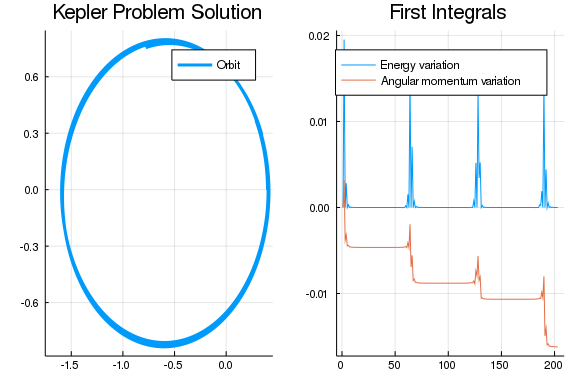There is almost no energy variation but angular momentum varies quite bit. How about only project to the angular momentum conservation manifold?

function angular_manifold(residual,u)
residual[1:2] .= initial_first_integrals - L(u[1:2], u[3:4])
residual[3:4] .= 0
end
angular_cb = ManifoldProjection(angular_manifold)
sol7 = solve(prob2, RK4(), dt=1//5, adaptive=false, callback=angular_cb)
analysis_plot2(sol7, H, L)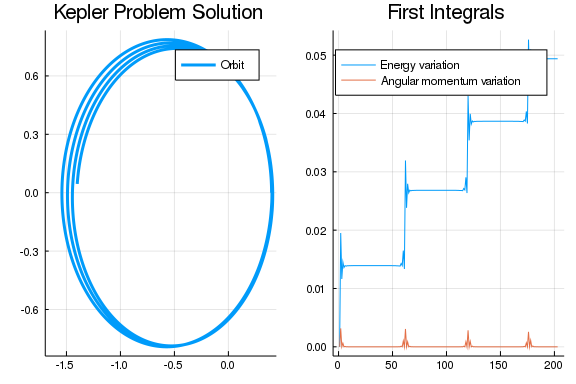Again, we see what we expect.

## Appendix

This tutorial is part of the DiffEqTutorials.jl repository, found at: https://github.com/JuliaDiffEq/DiffEqTutorials.jl

To locally run this tutorial, do the following commands:

using DiffEqTutorials
DiffEqTutorials.weave_file("models","05-kepler_problem.jmd")

Computer Information:

Julia Version 1.1.1
Commit 55e36cc308 (2019-05-16 04:10 UTC)
Platform Info:
OS: Linux (x86_64-pc-linux-gnu)
CPU: Intel(R) Core(TM) i7-3770 CPU @ 3.40GHz
WORD_SIZE: 64
LIBM: libopenlibm
LLVM: libLLVM-6.0.1 (ORCJIT, ivybridge)


Package Information:

Status ~/.julia/environments/v1.1/Project.toml
[7e558dbc-694d-5a72-987c-6f4ebed21442] ArbNumerics 0.5.4
[6e4b80f9-dd63-53aa-95a3-0cdb28fa8baf] BenchmarkTools 0.4.2
[be33ccc6-a3ff-5ff2-a52e-74243cff1e17] CUDAnative 2.2.0
[3a865a2d-5b23-5a0f-bc46-62713ec82fae] CuArrays 1.0.2
[55939f99-70c6-5e9b-8bb0-5071ed7d61fd] DecFP 0.4.8
[abce61dc-4473-55a0-ba07-351d65e31d42] Decimals 0.4.0
[ebbdde9d-f333-5424-9be2-dbf1e9acfb5e] DiffEqBayes 1.1.0
[eb300fae-53e8-50a0-950c-e21f52c2b7e0] DiffEqBiological 3.8.2
[459566f4-90b8-5000-8ac3-15dfb0a30def] DiffEqCallbacks 2.5.2
[f3b72e0c-5b89-59e1-b016-84e28bfd966d] DiffEqDevTools 2.9.0
[1130ab10-4a5a-5621-a13d-e4788d82bd4c] DiffEqParamEstim 1.6.0
[055956cb-9e8b-5191-98cc-73ae4a59e68a] DiffEqPhysics 3.1.0
[6d1b261a-3be8-11e9-3f2f-0b112a9a8436] DiffEqTutorials 0.1.0
[0c46a032-eb83-5123-abaf-570d42b7fbaa] DifferentialEquations 6.4.0
[31c24e10-a181-5473-b8eb-7969acd0382f] Distributions 0.20.0
[497a8b3b-efae-58df-a0af-a86822472b78] DoubleFloats 0.9.1
[f6369f11-7733-5829-9624-2563aa707210] ForwardDiff 0.10.3
[4138dd39-2aa7-5051-a626-17a0bb65d9c8] JLD 0.9.1
[23fbe1c1-3f47-55db-b15f-69d7ec21a316] Latexify 0.8.2
[eff96d63-e80a-5855-80a2-b1b0885c5ab7] Measurements 2.0.0
[961ee093-0014-501f-94e3-6117800e7a78] ModelingToolkit 0.2.0
[76087f3c-5699-56af-9a33-bf431cd00edd] NLopt 0.5.1
[2774e3e8-f4cf-5e23-947b-6d7e65073b56] NLsolve 4.0.0
[429524aa-4258-5aef-a3af-852621145aeb] Optim 0.18.1
[1dea7af3-3e70-54e6-95c3-0bf5283fa5ed] OrdinaryDiffEq 5.8.1
[65888b18-ceab-5e60-b2b9-181511a3b968] ParameterizedFunctions 4.1.1
[91a5bcdd-55d7-5caf-9e0b-520d859cae80] Plots 0.25.1
[d330b81b-6aea-500a-939a-2ce795aea3ee] PyPlot 2.8.1
[731186ca-8d62-57ce-b412-fbd966d074cd] RecursiveArrayTools 0.20.0
[90137ffa-7385-5640-81b9-e52037218182] StaticArrays 0.11.0
[f3b207a7-027a-5e70-b257-86293d7955fd] StatsPlots 0.11.0
[44cfe95a-1eb2-52ea-b672-e2afdf69b78f] Pkg